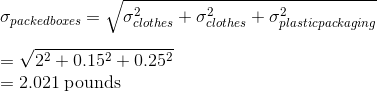# AP Statistics : How to find the standard deviation of the sum of independent random variables

## Example Questions

### Example Question #1 : How To Find The Standard Deviation Of The Sum Of Independent Random Variables

A high school calculus exam is administered to a group of students. Upon grading the exam, it was found that the mean score was 95 with a standard deviation of 12. If one student's z score is 1.10, what is the score that she received on her test?

110.1

107.2

109.2

108.2

105.3

108.2

Explanation:

The z-score equation is given as: z = (X - μ) / σ, where X is the value of the element, μ is the mean of the population, and σ is the standard deviation. To solve for the student's test score (X):

X = ( z * σ) + 95 = ( 1.10 * 12) + 95 = 108.2.

### Example Question #2 : How To Find The Standard Deviation Of The Sum Of Independent Random Variablesandare independent random variables. Ifhas a mean ofand standard deviation ofwhile variablehas a mean ofand a standard deviation of, what are the mean and standard deviation of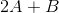?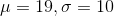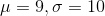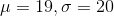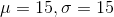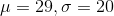Explanation:

First, find that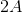has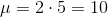and standard deviation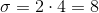.

Then find the mean and standard deviation of.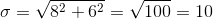### Example Question #3 : How To Find The Standard Deviation Of The Sum Of Independent Random Variables

Consider the discrete random variablethat takes the following values with the corresponding probabilities:

•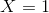with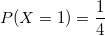•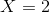with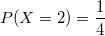•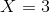with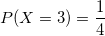•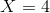with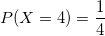Compute the variance of the distribution.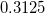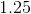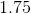Explanation:

The variance of a discrete random variable is computed as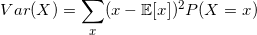for all the values ofthat the random variablecan take.

First, we compute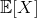, which is the expected value. In this case, it is.

So we have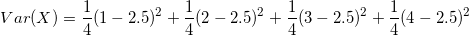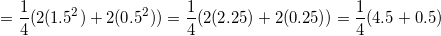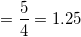### Example Question #1 : How To Find The Standard Deviation Of The Sum Of Independent Random Variables

Clothes 4 Kids uses standard boxes to ship their clothing orders and the mean weight of the clothing packed in the boxes ispounds. The standard deviation ispounds. The mean weight of the boxes ispound with a standard deviation of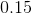pounds. The mean weight of the plastic packaging ispounds per box, with a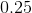pound standard deviation. What is the standard deviation of the weights of the packed boxes?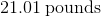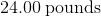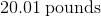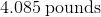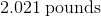Explanation:

Note that the weight of a packed box = weight of books + weight of box + weight of packing material used.

It is given that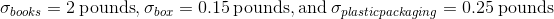.

The calculation of the standard deviation of the weights of the packed boxes is